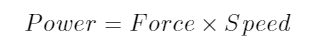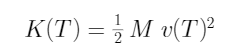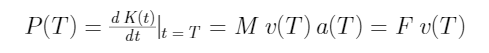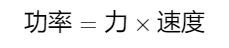# Supplementary Explanation on "Force-Power Equivalence“

Since the author recently published two blog posts

and

It has attracted the attention and discussion of many netizens. One of the key issues is the question of "the equivalence of force and power", that is, physics textbooks generally write the relationship between power and force as(1)

Indeed, it is an expression, but it is not an expression of "equivalence of force and power". Because the velocity on the right side of equation (1) can take any value, each velocity is related to the following special factors: the force environment, the physical process of the force, the mass of the force object, the resistance encountered, etc.  The equivalence relation pointed out by the author has nothing to do with these factors and is objective and direct.

For example, in an airplane traveling at 300 meters per second, a passenger pushes an object with a force F of 1 newton (equivalent to 1 watt of output power). Viewed from the ground, according to equation (1), the corresponding power is then equal to 300 watts. In fact, this is the result of the help of the plane.

To give another example, when t = 0, a fixed force of 1 Newton (equivalent to a power output of 1 watt) begins to push a primitive stationary object of mass M for uniform acceleration. When t = T, the speed of the object reaches v(T), according to the original physical meaning of formula (1): the work done by the force to the object during this time should be the kinetic energy K(T) obtained by the object,（2）

Using the usual relationship between power and kinetic energy, the power P(T)  reaches(3)

where a(T) is the acceleration (equal to a constant in the process). It is not difficult to see that equation (3) is equivalent to equation (2). Obviously, the power calculated in this way is related to the physical process. If you choose different time T, the power number P(T) obtained will be different. But the objective relationship, the force F = 1 Newton is equivalent to the power of 1 watt, has never changed!

Force is equivalent to Power（1）（2)(3)

https://blog.sciencenet.cn/blog-553379-1345731.html

## 全部精选博文导读

GMT+8, 2023-11-29 10:25